# Amateur Bands: A Top to Ten TRF

Since the mid-1930's - because of its far superior selectivity - the super-heterodyne principle has been the de-facto standard for practically all radio receivers. In certain very specific situations, however, the simplicity and passiveness of the TRF has distinct advantages. [PDF]

A super-heterodyne receiver contains an internal oscillator. This can introduce noise to the incoming signal and also cause the receiver to radiate a small signal, which could interfere with other equipment. A super-heterodyne is also vulnerable to strong "image" signals on the other side of the IFO (intermediate frequency off-set) oscillator. Weaker images can even occur at off-sets corresponding to multiples [or harmonics] of the IFO oscillator frequency.

There are also inherent tracking errors between the IFO oscillator and the received pass-band centre, which can effectively shift the resulting intermediate frequency, thereby attenuating signals differently at different parts of the tuning range. For higher frequencies, double conversion super-heterodynes are necessary, which can augment the tracking error and noise problems.

The use of an IF off-set beat-frequency oscillator [BFO], for hearing keyed carrier Morse Code transmissions, introduces yet more noise and can generate spurious harmonics between the BFO and the IFO oscillator.

A tuned radio frequency [TRF] receiver, on the other hand, is entirely passive in operation. It does not, within itself, generate any radio-frequency signals. It is there­fore much quieter [generates less spurious noise] than a super-heterodyne and tracking errors cannot exist. I have therefore opted for the much simpler TRF de­sign. Selectivity and audio shaping will be done using a comprehensive audio filter placed between the receiver's audio output and the final audio amplifier.

A variable capacitor with a capacitance ratio 3:4 facilitates a frequency ratio [bet­ween the bottom of its tuning range and the top of its tuning range] of about 1·15. Consequently, the ratio between the pass-band width at the low end of its tuning range and the pass-band width at the high end of its tuning range is also 1·15. So, if the pass-band at the low end of its tuning range be 10 kHz wide, then the pass-band at the high end of its tuning range will be 11·5 kHz wide. A constant pass-band width, of up to 10 kHz, for the receiver, across the whole of its tuning range, can easily be determined by a subsequent audio filter.

With this regime, a straight-forward TRF receiver can be more than adequately sel­ective for use on any of the amateur bands from Top to Ten. It renders the use of the super-heterodyne principle unnecessary.

A block diagram of the basic TRF receiver is shown below. The red line marks the boundary between what I call the Front End and what I call the Back End. The Front End deals with the RF signal up to its detection: the extraction of the audio content. The Back End deals with shaping and amplifying the audio content. I propose that there be a separate instance of the Front End for each of the Amateur Bands, which share, in turn, a single instance of the Back End. Different Front Ends may contain different numbers of RF stages to achieve necessary RF selectivity.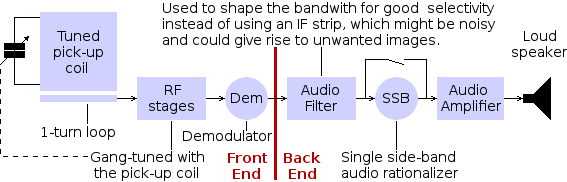I first consider the Front End, beginning with my preferred design for the signal pick-up coil and RF stage tuning coils for each Amateur Bands from Top to Ten.

### Pick-up and Tuning Coils

To provide a well-defined low-noise pass-band, I have opted not to use an aerial wire or any form of rod antenna to pick up the arriving signal. Instead, I have decided to use a very large and very high-Q coil to capture the magnetic vector of the arriving signal. The magnetic vector of the arriving signal is also less prone to artificially generated radio interference. This coil is to be tuned using a section of the multi-gang variable capacitor used to tune the radio-frequency stages of the receiver. The fact that the signal pick-up device is variably tuned means that a narrower lower-noise signal is supplied to the input of the receiver.The pick-up coil should be mounted with its axis vertical. This will cause it to pick up signals that are horizontally polarized elec­trically, which is correct for practically all transmissions on these bands. It will have a good omni-directional horizontal gain. Its tuning capacitor should be mounted quite close to it, being var­ied via a tort cord and pulley system ganged to the receiver's tuning capacitors. Alternatively, the capacitor could be varied via a synchro motor linked to a synchro generator mounted on the receiver's main tuning shaft. The pick-up coil can be mounted inside a building but it should be mounted as high as practicable, away from steel-reinforced walls or beams. The illustration on the right shows a mast-mounted version of a pick-up coil for Top Band [160 metres: 1695 to 1957 kHz]. The 10-metre mast is a hollow perspex tube 50mm diameter. It is kept vertical by three guy ropes running 20° from the vertical and spaced 120° apart around the mast. Each guy is fixed to an anchor half-way up the mast. At this half-way point are three horizontal radials of 13mm perspex tube also spaced 120° apart but off-set from the guys by 60°. These act as spacers for three pole-guys, whose purpose is to keep the whole of the mast straight. The top short cylinder is the pick-up coil. The lower one houses the tuning control servo and feeder terminator.

The tuned circuits for the RF stages of the receiver use smaller coils with the same inductance as the pick-up coil. The parameters and dimensions for the pick-up and tuning coils for the HF Amateur Radio Bands are shown below.

These values were calculated using Calculator 1, which opens in a separate tab. This calculates a coil's inductance, the top frequency of the desired tuning range, the coil's radius, its number of turns, its diameter [=length] and the amount of wire required from the minimum frequency of the tuning range, the maximum and min­imum of the variable capacitor and the desired coil pitch. The length and diameter of the coil are made equal because, in practice, this results in the highest Q-factor [inductor with minimum inherent capacitance]. Alternatively, if you prefer to specify the coil's diameter [=length], pitch and tuning range, then use Calculator 2, which also opens in a separate tab.

For best results, all coils should be wound with Litzwire, which comprises a number of separately insulated strands of fine copper or silver wire plaited into a light com­posite cable.

### Coils For The Amateur 2200 Metre Band: 135.7 to 137.8 kHz kHz

Frequency ratio of coil: 1·7320508075688774

Use tuning capacitor range 50 to 150 pF
Minimum frequency, Fmin = 100 kHz
Inductance needed to tune 100000 Hz with 50 pF capacitance:
L = 0·016886863935979695 Henries.
Maximum frequency attainable with 150 pF capacitor:
Fmax = 173205·08075688774 Hertz

Pick-up Coil
Make the pitch, P = 0·02 metres per turn.
Coil radius, R = 1·0751662077787074 metres
Coil winding, N = 107·51662077787073 turns
Length=diameter, D = 0.85335998463429 metres
Wire required, W = 726.3283301737007 metres

Tuning Coil
Make the pitch, P = 0·005 metres per turn
Coil radius, R = 0·426679992317145 metres
Coil winding, N = 170·671996926858 turns
Length=diameter, D = 0·85335998463429 metres
Wire required, W = 457·55696671220716 metres

From the point of view of practicality, I think this coil aerial should be mounted with its central axis vertical, giving a horizontal electric vector and a vertical magnetic vector. However, from the point of view of propagation, I haven't a clue which is best, especially from the point of view of ground absorption. I think the best con­struction would be to channel the litz wire of the coil down a coiled mica tube sup­ported on a cylindrical plastic frame former.

### Coils For The Amateur Top Band: 1695 to 1957 kHz

Frequency ratio: 1·154572271

Use tuning capacitor range 375 to 500 pF
Minimum frequency, Fmin = 1695 kHz
Inductance needed to tune 1695000 Hz with 500 pF capacitance:
L = 0·000017633188645396083 Henries.
Maximum frequency attainable with 375 pF capacitor:
Fmax = 1957217·4125528312 Hertz

Pick-up Coil
Make the pitch, P = 0·02 metres per turn.
Coil radius, R = 0·1090777629951081 metres
Coil winding, N = 10·90777629951081 turns
Length=diameter, D = 0·2181555259902162 metres
Wire required, W = 7·47889015033212 metres

Tuning Coil
Make the pitch, P = 0·005 metres per turn
Coil radius, R = 0·04328753893119314 metres
Coil winding, N = 17·31501557247725 turns
Length=diameter, D = 0·1175004582538685 metres
Wire required, W = 4·71019647271448 metres

The axis of this aerial coil must be vertical.

### Coils For The 80-Metre Amateur Band: 3·5 to 4·0 MHz

Frequency ratio: 1·142857143

Use a 90 to 120 pF 3-gang variable capacitor.
Minimum frequency, Fmin = 3·5 kHz
Inductance needed to tune Fmin with 120 pF capacitance:
L = 0·00001723149381222418 Henries.
Maximum frequency attainable with 90 pF capacitor:
Fmax = 4041451·8843273805 Hertz

Pick-up Coil
Make the pitch, P = 0·02 metres per turn.
Coil radius, R = 0·1082431064691129 metres
Coil winding, N = 10·82431064691129 turns
Length=diameter, D = 0·2164862129382258 metres
Wire required, W = 7·364920521041236 metres

Tuning Coil
Make the pitch, P = 0·005 metres per turn.
Coil radius, R = 0·04295630526934391 metres
Coil winding, N = 17·18252210773756 turns
Length=diameter, D = 0·08591261053868782 metres
Wire required, W = 4·63840011078122 metres

It picks up the signal's magnetic vector, so it can be placed inside a brick building provided the walls contain no magnetic materials (iron, nickel or cobalt).

### Coils For The 40-Metre Amateur Band: 6·5 to 7·5 kHz

Frequency ratio: 1·153846154

Use a 27 to 36 pF 3-gang variable capacitor.
Minimum frequency, Fmin = 6·5 kHz
Inductance needed to tune Fmin with 36 pF capacitance:
L = 0·00001665371196842179 Henries.
Maximum frequency attainable with 27 pF capacitor:
Fmax = 7505553·499465135 Hertz

Pick-up Coil
Make the pitch, P = 0·02 metres per turn.
Coil radius, R = 0·10701950908942125 metres
Coil winding, N = 10·701950908942123 turns
Length=diameter, D = 0·2140390181788425 metres
Wire required, W = 7·199424688850118 metres

Tuning Coil
Make the pitch, P = 0·005 metres per turn.
Coil radius, R = 0·08834265830642155 metres
Coil winding, N = 35·33706332256862 turns
Length=diameter, D = 0·1766853166128431 metres
Wire required, W = 22·47302633266358 metres

The axis of this aerial coil must be vertical.

### Coils For The 20-Metre Amateur Band: 13 to 15 MHz

Frequency ratio: 1·153846154

Use a 7·5 to 10 pF 3-gang variable capacitor.
Minimum frequency, Fmin = 13 MHz
Inductance needed to tune 13 MHz with 7·5 pF capacitance:
L = 0·000014988340771579611 Henries.
Maximum frequency attainable with 10 pF capacitor:
Fmax = 15011106·99893027 Hertz

Pick-up Coil
Make the pitch, P = 0·02 metres per turn.
Coil radius, R = 0·14023522872928076 metres
Coil winding, N = 14·023522872928075 turns
Length=diameter, D = 0·28047045745856153 metres
Wire required, W = 10·053125844402867 metres

Tuning Coil
Make the pitch, P = 0·005 metres per turn.
Coil radius, R = 0·04100502963267555 metres
Coil winding, N = 16·402011853070217 turns
Length=diameter, D = 0·0820100592653511 metres
Wire required, W = 4·2266461138243665 metres

The axis of the aerial coil is vertical, so the polarization of the aerial is horizontal, which is normal for this band.

### Coils For The 15-Metre Amateur Band: 20 to 23 MHz

Frequency ratio: 1·15

Use a 3 to 4 pF 3-gang variable capacitor.
Minimum frequency, Fmin = 20 MHz
Inductance needed to tune 20 MHz with 4 pF capacitance:
L = 0·000015831434939980964 Henries.
Maximum frequency attainable with 3 pF capacitor:
Fmax = 23094010·767585028 Hertz [23 MHz]

Pick-up Coil
Make the pitch, P = 0·02 metres per turn.
Coil radius, R = 0·10522833489277751 metres
Coil winding, N = 10·522833489277751 turns
Length=diameter = 0·21045666978555502 metres
Wire required, W = 6·960555010955296 metres

Tuning Coil
Make the pitch, P = 0·005 metres per turn.
Coil radius, R = 0·041759892376414254 metres
Coil winding, N = 16·7039569505657 turns
Length=diameter = 0·08351978475282851 metres
Wire required, W = 4·38366582298374 metres

The axis of the aerial coil is vertical, so the polarization of the aerial is horizontal, which is normal for this band.

### Coils For The 10-Metre Amateur Band: 27·5 to 30·5 MHz

Frequency ratio: 1·109090909

Use a 2 to 2·5 pF 3-gang variable capacitor.
Minimum frequency, Fmin = 28 MHz
Inductance needed to tune Fmin with 2·5 pF capacitance:
L = 0·000013397842461603724 Henries.
Maximum frequency attainable with 2 pF capacitor:
Fmax = 30745934·690622106 Hertz [30·7 MHz]

Pick-up Coil
Make the pitch, P = 0·02 metres per turn.
Coil radius, R = 0·08510026246010823 metres
Coil winding, N = 8·510026246010822 turns
Length = Diameter = 0·17020052492021645 metres
Wire required, W = 4·553499137014897 metres

Tuning Coil
Make the pitch, P = 0·005 metres per turn.
Coil radius, R = 0·03377206153798642 metres
Coil winding, N = 13·508824615194564 turns
Length = Diameter = 0·06754412307597284 metres
Wire required, W = 2·867315845231706 metres

The axis of the aerial coil is vertical, so the polarization of the aerial is horizontal, which is normal for this band.

I have omitted the 30, 17 and 12 metre Amateur Bands because these were alloc­ated much later and are not so well used. You can use my calculators to calculate the specifications of the coils required for these bands if you wish. The minimum to maximum frequency ratio of the short-wave broadcast bands is of the same order as those of the amateur bands. The same TRF technique can therefore be succe­ssfully employed for receiving broadcasts from around the world on these bands too. Coils for the short-wave broadcast bands can also be specified using my coil calculators.

### The Front End

A more detailed schematic of the Front End is shown below. Each RF resonator (pick-up or tuning coil with its respective variable capacitor) is electrically isolated. It is coupled only inductively to the rest of the receiver. In the case of the pick-up coil, the signal is picked up from the resonator by a single turn of Litz wire wound on one end of the coil former. Each tuning coil has a loop comprising a few turns of wire wound at each end of the main resonator coil. One loop is to allow the input signal to energize the resonator. The other is to pick up the output signal from the reson­ator.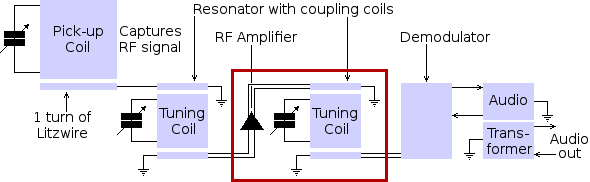An RF amplifier then strengthens the signal and passes it to the next resonator. I have shown only two resonator stages. More can be added as required to achieve the desired pass-band width at the low-frequency end of the tuning range. Reson­ator stages are added by repeating the detail within the red rectangle above. The resonators of the different stages can be off-set one side or the other of the pick-up resonator's frequency in order to flatten the top of the pass-band profile. The final resonator feeds the signal to the demodulator.The demodulator extracts the audio frequency content of the original RF signal. The RF signal is taken from the final RF resonator via a small in­ductive coupling coil comprising a few turns of Litz wire wound at one end. The coupling coil is earthed at its mid point to provide a balanced source. Opposing diodes separate the +ve and −ve halves of the RF signal. Their outputs are fed to the balanced input of an audio transformer with a centre-tapped primary. The inductance of the audio transformer's primary winding is well high enough to block the RF components of the signal, thus allowing only the audio content to pass through to the output winding.

I prefer the centre-tapped transformer configuration with just two diodes because this allows both the radio and audio frequency signals to be balanced with respect to chassis potential (earth or ground). Impedance calculations should take account that each half-cycle at radio frequency uses only half of the radio frequency coupling coil's winding and that likewise, each half-cycle at audio frequency uses only half of the primary winding of the audio transformer. I also prefer to earth (ground) the centre tap of the audio transformer output winding to balance the audio signal with respect to chassis, thus minimizing its susceptibility to electrical interference on its way to the receiver's Back End, which is in a separate cabinet.

If amplification is necessary at this stage, the opposing diodes could be replaced by op-amps configured for +ve and −ve inputs and outputs respectively. Forming a Morse Code tone from a keyed continuous wave transmission or reconstituting the speech from a single-sideband transmission is done later in the Back End of the receiver. I think it must be nostalgia that makes me yearn to implement the RF am­plifiers herein using electro-thermionic triodes. Notwithstanding, I think I will always end up using packaged op-amps.

### The Back End

There is a separate Front End for each radio band. These all share just one Back End. A block schematic of the Back End is shown below.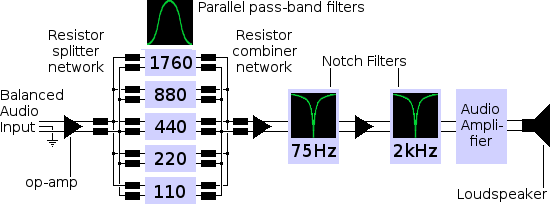The Back End receives, from the Front End to which it is currently connected, a balanced audio signal. Because of the 1:1·15 frequency ratio of the Front End's pass-band between the lower and upper ends of its tuning range, the pass-band of the audio signal presented to the Back End will vary by the same factor of 1:1·15. The function of the Back End is firstly to make the audio pass-band constant over the whole tuning range. It achieves this objective by allowing only 5 octaves of the audio spectrum to pass through to the audio amplifier and loudspeaker. The highest unattenuated frequency allowed through is thus 16 times the lowest unattenuated frequency allowed through. Each of the 5 permitted octaves is allowed to pass through its own one-octave wide tuned filter. This requires 5 different and separate one-octave filters wired in parallel, as shown in the diagram above.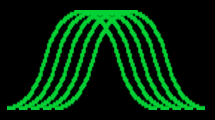The centre frequencies chosen for the 5 parallel ac­ceptance filters are 110, 220, 440, 880 and 1760 Hertz. The trace on the left shows the 5 overlapping accept­ance bands peaked one octave apart. The horizontal axis shows frequency according to a logarithmic scale. The vertical axis shows the ampli­tude of the signal al­lowed through.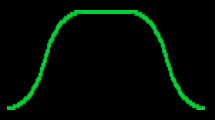The pass-band profile produced by combining the out­puts of the 5 acceptance filters is shown on the right. This now has the correct 5-octave bandwidth. How­ever, its sides are not yet sufficiently steep. In other words, the degree to which it rejects all frequencies above and below the desired pass-band is not yet ad­equate.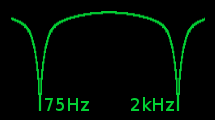For this reason, the signal, gained by merging the outputs of the 5 parallel acceptance filters, is amplified and then passed through two successive rejection (or notch) filters. Each of these strongly rejects signals at frequencies at – and close to – its tuning point (75Hz & 2kHz respectively), as shown in the trace on the left.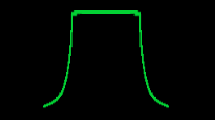This causes a steep attenuation of the audio signal at the upper and lower boundaries of the desired five octave frequency range. The resulting pass-band now approximates much more closely to the ideal square all-or nothing profile, as shown on the right. The output signal from the second notch filter is then passed on to a good quality audio amplifier.

### The Acceptance Filters

I have decided to use 5 inductance-capacitance filters whose centre frequencies correspond to the A-note in each of 5 octaves of the piano. Middle-A on the piano has a frequency of 440 hertz. Each A-note going down the keyboard has half the frequency of the previous A-note. Each A-note going up the keyboard has double the frequency of the previous A-note. The centre frequencies for my 5 pass-band filters are therefore 110, 220, 440, 880, 1760 hertz.A diagram of an LC (inductance-capacitance) pass-band filter is shown on the right. The inductive re­actance of the coil, XL=2πfL, where f is the frequ­ency of the presented signal and L is the value of its inductor in henries. The capacitive reactance of the capacitor, XC=1/(2πfC), where C is the value of the capacitor in farads. The filter passes the in­coming signal with least opposition when XC = XL.

The following calculator calculates the required inductance value in henries for a pass-band filter of a given centre-frequency and standard capacitor value. Type in your values for frequency and capacitance and press the carriage-return key within either of these fields. The required inductance appears in the third (bottom) field. The default values shown are for a filter which will allow through signals that fall within the octave centred on Middle-A [440 Hz].

Required Inductance, L Pass-band Frequency, Fmin Hertz Chosen Capacitance, Cmax Farads

1100.00001000.209
2200.00001000.052
4400.00000100.131
8800.00000100.033
17600.00000010.082
The table on the left shows the values thus calculated for the 5 filters I need to limit my audio pass-band to frequencies which fall only within the 5 octaves I requ­ire. However, the calculations have given some awk­ward values for the inductances required. I would like to see how far the centre frequencies of my filters will be perturbed if I use the nearest standard inductor values.

The following calculator takes the standard capacitor value from the previous cal­culator and calculates the filter's centre frequency from an entered inductor value. So, if I use a 130 millihenry inductor instead of the 130·8383053310797 millihenry inductor value given by the first calculator, I get a centre-frequency value of 441·4163908290642 hertz instead of 440 hertz. I can live with that.

Centre Frequency, Fmin Hertz

0.00001000.200 113
0.00001000.050 225
0.00000100.130 441
0.00000100.030 919
0.00000010.0801779
The table on the right gives the centre-frequencies of my 5 filters where I am using more rounded values for the inductances. Of these, the worst is only about 4% out, which is somewhere in between the notes A and A#. I don't think that this could make any per­ceptible difference to the range of frequencies which will ultimately arrive at the list­ener's ears.Each of the five pass-band filters must pass its respective octave of the audio signal. This necessitates that the 5 pass-band filters must operate in parallel. The output from the Back End's first op-amp must therefore be split 5 ways to provide a separate independent input for each filter. This splitting is done by what is, in effect, a 6-way star network of resistors, as shown on the left.

The 6 ways comprise one input and 5 outputs. The value, R, of each resistor must be one sixth the operating impedance of the op-amp and the filters. There must be a separate 5-way splitter for each of the two outputs from the balanced op-amp. Each pass-band filter thus comprises two identical instances of the following circuit.The values shown are for the 440 Hz filter. The four resistors, R, all have the same value in all filters, equal to one sixth of the impedance of the op-amp's outputs. The two resistors on the left are each part of a separate 6-way star splitter. The two resistors on the right are each part of a separate 6-way star signal merger, which merges the outputs of the 5 pass-band filters ready for input to the Back End's second op-amp. Ideally, I would include an op-amp in each filter, as show below.I would make the gain of the op-amp manually adjustable by means of a sliding variable resistor. This would allow me to even out any irregularity in the pass-band and also reduce the bandwidth in the presence of troublesome higher octave inter­ference.

### Notch Filters

The upper and lower edges of the composite pass-band of the 5 filters so far de­scribed are not steep enough to completely avoid interference on frequencies just outside the pass-band. I shall therefore steepen these edges by placing notch rejec­tion filters, one either side of the pass-band, as shown in the following graph.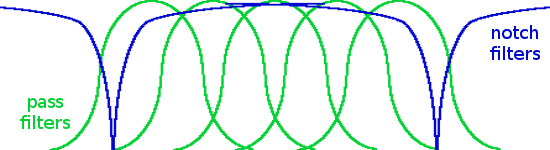All these signals should add up to a reasonably steep-sided rectangular pass-band.A circuit diagram of the generic LC (inductance-capacitance) rejection (or notch) filter is shown on the right. The relationship between resonant frequ­ency, capacitance and inductance is the same as for an acceptance (or pass-band) filter. The same calculators, as before, can therefore be used also to calculate the values of the components (induc­tor and capacitor) required for the Back End's re­jection filters.

Recap: The coil's inductive reactance, XL = 2πfL, where f is the frequency of the presented signal and L is the coil's inductance in henries. The capacitor's capacitive reactance, XC = 1/(2πfC), where C is its capacitance in farads. The filter rejects the incoming signal with maximum opposition when XC = XL. The values shown in the above diagram are for a 75Hz rejection filter. This rejects (does not allow to pass through) signals with frequencies at and around 75 Hz.

The two rejection (notch) filter stages of the Back End are shown in the following diagram. These stages form a balanced two-channel system, using two-channel differential op-amps. One channel carries the positive half-cycles of the audio signal while the other channel carries the negative half-cycles of the audio signal. Since there are two stages with two channels, four notch filters are required, each shown in a red square. Thus there are two 75Hz filters and two 2kHz filters.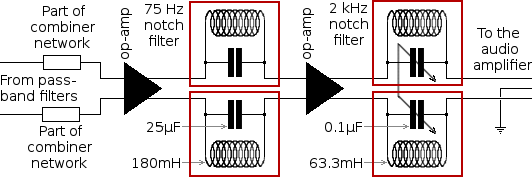I have used variable capacitors in the 2kHz notch filters. These variable capacitors are ganged. This allows me to move the notch frequency in case I need to eliminate a troublesome heterodyne on any particular frequency. The 0·1μF capacitance can be made up, in practice, of a lower value variable capacitor with an additional fixed capacitor.

The output from the second notch filter is passed - via a balanced feed - to a good quality audio amplifier and loudspeaker.